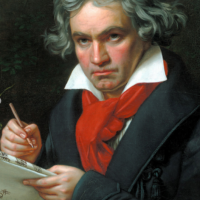# Math Club (May) : Fibonacci Numbers (Patterns, Puzzles and Geometry)

• ##### Week 1

Problems with Fibonacci series

• ##### Week 2

Experiments with Fibonacci series

• ##### Week 3

Previous Challenges and a strategy game!

• ##### Week 4

The Chocolate division and Nim Strategy Game

## Math Club (May) : Fibonacci Numbers (Patterns, Puzzles and Geometry)

### Challenge 1

We performed around 4 experiments with the Fibonacci series. Describe one of your favourite experiment. What did we discover?

PS: You can go through the recording again.

## 2 thoughts on “Challenge 1”

1.Sir, I especially loved the experiment where we squared two consecutive Fibonacci numbers, and added them together to get the next Fibonacci number.

2.sir
we did square numbers of fibonacci numbers.
after that we added consecutive two fibonacci numbers
result- we found alternate fibonacci numbers
that was interesting

i wish to find out more about it why it exist in nature as well